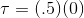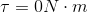# SAT II Physics : Forces

## Example Questions

### Example Question #1 : Frictional Force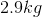crate slides across a floor for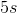before coming to rest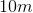from its original position.

What is the force due to friction?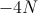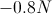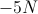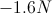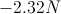Explanation:

Since there is only one force acting upon the object, the force due to friction, we can find its value using the equation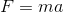. The problem gives us the mass of the crate, but we have to solve for the acceleration.

Start by finding the initial velocity. The problem gives us distance, final velocity, and change in time. We can use these values in the equation below to solve for the initial velocity.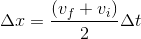Plug in our given values and solve.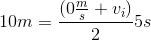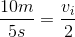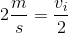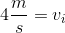We can use a linear motion equation to solve for the acceleration, using the velocity we just found. We now have the distance, time, and initial velocity.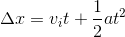Plug in the given values to solve for acceleration.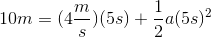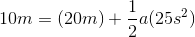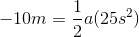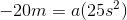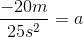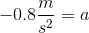Now that we have the acceleration and the mass, we can solve for the force of friction.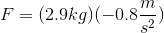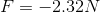### Example Question #11 : Mechanics

If the distance between two objects is reduced by two-thirds, how will the gravitational force between the objects be affected?

Increases by a factor of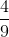Decreases by a factor ofIncreases by a factor ofIncreases by a factor ofDecreases by a factor ofIncreases by a factor ofExplanation:

According to Newton's law of universal gravitation, the gravitational force between two objects is inversely proportional to the square of the distance between them.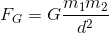If the distance is reduced by two-thirds, then the final distance is equal to one-third the original distance.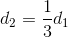Using this term in the equation will show that the force increases by a factor of nine.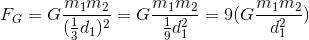### Example Question #1 : Forces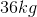girl bounces on a massless pogo stick. If the spring constant for the stick is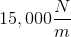, what is the maximum compression of the spring?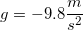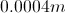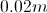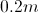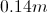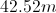Explanation:

There are two forces at work here: the force due to gravity and the restoring force of the spring. We can set these two forces equal to one another because the forces must be in equilibrium when the spring is compressed at its maximum point.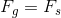Expand this equation by using the formulas for gravitational and spring force, respectively.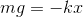Plug in our given values for the girl's mass, gravitational acceleration, and the spring constant. Using these values, we can solve for the displacement of the spring.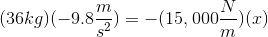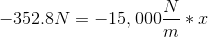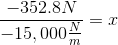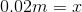### Example Question #1 : Forces

A certain spring has an equilibrium length of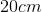. When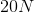of force are applied, the spring length becomes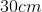. What is the spring constant?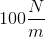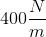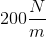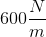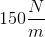Explanation:

The equation for spring force is: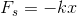The force applied to the spring will be equal and opposite the force of the spring itself. In this case, the force applied is, so the force of the spring will be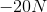.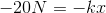The change in distance will be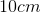, or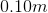. Using this value, we can solve for the spring constant.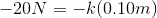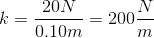### Example Question #1 : Other Mechanical Forces

A wrench is being used to tighten a bolt. The center of the bolt is the pivot point, and the wrench is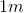long. If a force of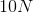is applied at the midpoint of the wrench and that force is parallel to the wrench, what is the torque?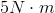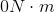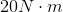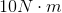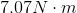Explanation:

The equation for torque is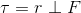The force is applied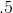meters from the pivot. and in this case because the force is parallel, the perpendicular component of force is zero, so: